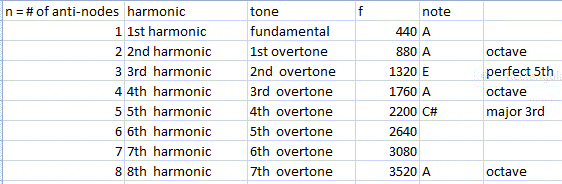## Sound waves

Sound waves are longitudinal waves and travel by compressing (and causing rarefactions of) the medium through which they travel.  Despite many great Hollywood science fiction battles, “In space no one can hear you scream“, because there is no (well almost no) medium for sound waves to travel through.

# Pitch

The pitch that we hear depends on the frequency of the sound wave.  The normal range of human hearing of a young person is 20 Hz to 20,000 Hz.  In class, you are verifying this often cited claim.   Other animals can hear below and above this range.  What do sounds below this range sound like? What is your maximum and minimum range?

# Loudness

The amplitude of the sound wave corresponds to the loudness of the wave.  Normally, when we think of amplitude we think of the height of the crests and troughs in a wave.  However, since we are dealing with longitudinal waves, we are working with the amplitude of the compression.  The units for loudness are often given in dB or Decibels, where the Decibel is actually 1/10th of a Bel which is named after the famous Boston University Professor, Alexander Graham Bell. Graham was a professor in the school of Oratory, and taught elocution. The decibel scale is actually a logarithmic scale, not a linear scale.  So if the amplitude increases by a factor of 10, it is 10 dB greater.  If the amplitude increases by a factor of 100, it is 20dB greater, 1000 it is 30dB greater.

We often look at sound waves with electronic equipment such as MyDAQ.  We used MyDAQ in class today to generate sine waves using the Function Generator. However, when we look at the waves on an oscilloscope, the appear to be transverse as that is easier for us to understand.  Additionally, a microphone will convert the longitudinal sound waves into transverse waves of electrical current.# Tone

You might also notice that the Function generator allows you to generate sine waves, triangle waves, and square waves.  The sine wave is one of the simplest waves produced in nature.  A tuning fork actually produces a sine wave which has a very simple and bland tone.  More complicated instruments produce sounds with a more complicated tone (or timbre) or wave shape.  These more complicated waves are actually a combination of dozens of sine waves.  A guitar string or a trumpet is simultaneously vibrating with all these harmonics (and each harmonic itself is a sine wave).  The interference of all these waves produces a rich tone, and a complex wave shape.

# Music

There are intricate mathematical relationships between the musical notes.  There are mathematical reasons that certain combinations of notes sounds pleasing to our ears (consonance) and certain combinations sound displeasing (dissonance).

## Octave

The octave by definition is a doubling of the frequency of a note.  For instance, the standard frequency for the musical note A is 440 Hz.  One octave up would be another A, and this would be at 880 Hz.  One more octave would be another doubling or 1760 Hz.  Another octave up would be 3520 Hz.  An octave below the original 440 Hz would be at 220 Hz, 110 Hz, and 55 Hz, which are all A on the scale.

## Major 3rd

If we were to multiply our original frequency, 440 Hz by a factor of 5, then we would have

f =  5 * 440Hz = 2200 Hz

This is what we call a Major 3rd.  In the case of an A note, the Major 3rd is a C #, or at 550 Hz.

## Perfect 5th

If we were to multiple our original frequency, 440 Hz by a factor of 3, then we would have

f = 3 * 440Hz = 1320 Hz

This note is a Perfect fifth.  The E above an A is at 660 Hz, and one octave above that E is 1320 Hz.

## Harmonics Overtones

So we have several vernacular when talking about overtones and Harmonics.

The 1st harmonic, or fundamental tone generally has only one Anti-node or region where there is constructive interference.

The 2nd harmonic is the first tone OVER the fundamental, thus often called the 1st overtone.

For a string or an open pipe we would haveIn a closed pipe however, remember that the number of anti-nodes in a harmonics increases with odd integers.

Up Next: 2D Interference, Dead Spots, and Beats, Wave Shape